## Derivative

The derivative of a Function represents an infinitesimal change in the function with respect to whatever parameters it may have. The simple'' derivative of a function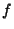with respect tois denoted eitheror(and often written in-line as). When derivatives are taken with respect to time, they are often denoted using Newton'sFluxion notation,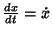. The derivative of a function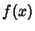with respect to the variableis defined as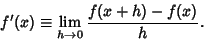(1)

Note that in order for the limit to exist, both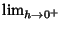and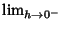must exist and be equal, so the Function must be continuous. However, continuity is a Necessary but not Sufficient condition for differentiability. Since some Discontinuous functions can be integrated, in a sense there are more'' functions which can be integrated than differentiated. In a letter to Stieltjes, Hermitewrote, I recoil with dismay and horror at this lamentable plague of functions which do not have derivatives.''

A 3-D generalization of the derivative to an arbitrary direction is known as the Directional Derivative. In general, derivatives are mathematical objects which exist between smooth functions on manifolds. In this formalism, derivatives are usually assembled into Tangent Maps.''

Simple derivatives of some simple functions follow.(2)(3)(4)(5)(6)(7)(8)(9)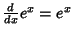(10)(11)(12)(13)(14)(15)(16)(17)(18)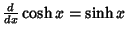(19)(20)(21)(22)(23)(24)(25)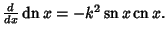(26)

Derivatives of sums are equal to the sum of derivatives so that(27)

In addition, if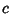is a constant,(28)

Furthermore,(29)

where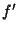denotes the Derivative ofwith respect to. This derivative rule can be applied iteratively to yield derivate rules for products of three or more functions, for example,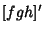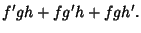(30)

Other rules involving derivatives include the Chain Rule, Power Rule, Product Rule, and Quotient Rule. Miscellaneous other derivative identities include(31)(32)

If, whereis a constant, then(33)

so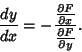(34)

A vector derivative of a vector function(35)

can be defined by(36)

See also Blancmange Function, Carathéodory Derivative, Comma Derivative, Convective Derivative, Covariant Derivative, Directional Derivative, Euler-Lagrange Derivative, Fluxion, Fractional Calculus, Fréchet Derivative, Lagrangian Derivative, Lie Derivative, Power Rule, Schwarzian Derivative, Semicolon Derivative, Weierstraß Function

References

Abramowitz, M. and Stegun, C. A. (Eds.). Handbook of Mathematical Functions with Formulas, Graphs, and Mathematical Tables, 9th printing. New York: Dover, p. 11, 1972.

Anton, H. Calculus with Analytic Geometry, 5th ed. New York: Wiley, 1987.

Beyer, W. H. Derivatives.'' CRC Standard Mathematical Tables, 28th ed. Boca Raton, FL: CRC Press, pp. 229-232, 1987.